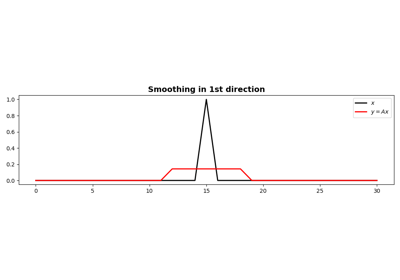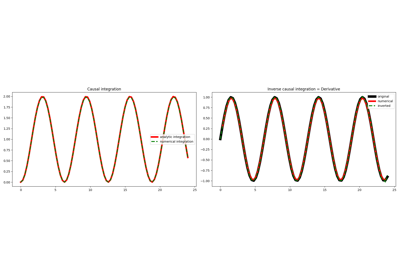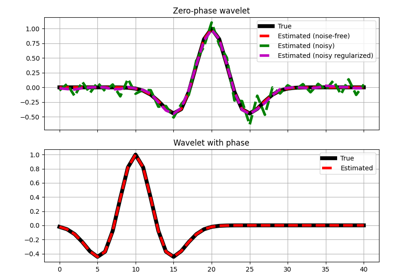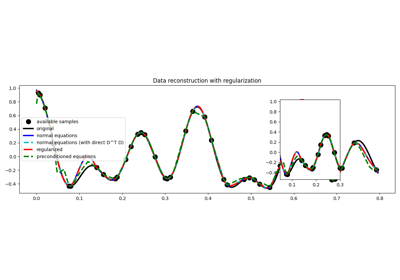# pylops.Smoothing1D¶

pylops.Smoothing1D(nsmooth, dims, axis=-1, dtype='float64')[source]

1D Smoothing.

Apply smoothing to model (and data) to a multi-dimensional array along axis.

Parameters
nsmoothint

Length of smoothing operator (must be odd)

dims

Number of samples for each dimension

axisint, optional

New in version 2.0.0.

Axis along which model (and data) are smoothed.

dtypestr, optional

Type of elements in input array.

Notes

The Smoothing1D operator is a special type of convolutional operator that convolves the input model (or data) with a constant filter of size $$n_\text{smooth}$$:

$\mathbf{f} = [ 1/n_\text{smooth}, 1/n_\text{smooth}, ..., 1/n_\text{smooth} ]$

When applied to the first direction:

$y[i,j,k] = 1/n_\text{smooth} \sum_{l=-(n_\text{smooth}-1)/2}^{(n_\text{smooth}-1)/2} x[l,j,k]$

Similarly when applied to the second direction:

$y[i,j,k] = 1/n_\text{smooth} \sum_{l=-(n_\text{smooth}-1)/2}^{(n_\text{smooth}-1)/2} x[i,l,k]$

and the third direction:

$y[i,j,k] = 1/n_\text{smooth} \sum_{l=-(n_\text{smooth}-1)/2}^{(n_\text{smooth}-1)/2} x[i,j,l]$

Note that since the filter is symmetrical, the Smoothing1D operator is self-adjoint.

Attributes
shapetuple

Operator shape

explicitbool

Operator contains a matrix that can be solved explicitly (True) or not (False)

## Examples using pylops.Smoothing1D¶1D Smoothing

1D SmoothingCausal Integration

Causal IntegrationWavelet estimation

Wavelet estimation03. Solvers

03. Solvers